/

### Function Description

Returns a vector containing the ‘implied alphas’ for a given set of active positions, i.e. the return assumptions that need to be held for a portfolio to be optimal (ignoring constraints), given active positions, standard deviations, a correlation matrix and a trade-off factor (i.e. risk aversion factor) that corresponds to the investor’s chosen trade-off between return and risk. It is assumed that the investor has a quadratic utility function of the following form, whereis a vector of returns,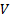is a covariance matrix and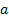is a vector of active weights (i.e.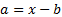, where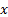is a vector of portfolio weights withelements and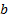is the corresponding vector of benchmark weights):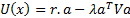Please bear in mind that if a given set of returns,, is optimal in this context then so is the set of returns defined by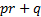for any constant (scalar, i.e. asset class independent) values of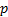and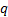. This function adopts the convention that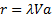or equivalently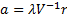, i.e. that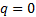and thatscales in line with the risk-return trade-off factor, i.e.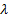.

Please also bear in mind that if the active position within a live portfolio is at the limit of a constraint (e.g. for a long-only portfolio the portfolio weight is zero, i.e. constrained by the long-only constraint) then it is not possible to calculate accurately the implied alpha for that position, since we do not then know (merely from the portfolio weights) how positive or negative is the view that the manager is assigning to that position.

Contents | Prev | Next